Courses

# CBSE Mathematics 2017 - Final Preparation (Score Predictor Test)

## 45 Questions MCQ Test Additional Question Bank | CBSE Mathematics 2017 - Final Preparation (Score Predictor Test)

Description
This mock test of CBSE Mathematics 2017 - Final Preparation (Score Predictor Test) for Class 10 helps you for every Class 10 entrance exam. This contains 45 Multiple Choice Questions for Class 10 CBSE Mathematics 2017 - Final Preparation (Score Predictor Test) (mcq) to study with solutions a complete question bank. The solved questions answers in this CBSE Mathematics 2017 - Final Preparation (Score Predictor Test) quiz give you a good mix of easy questions and tough questions. Class 10 students definitely take this CBSE Mathematics 2017 - Final Preparation (Score Predictor Test) exercise for a better result in the exam. You can find other CBSE Mathematics 2017 - Final Preparation (Score Predictor Test) extra questions, long questions & short questions for Class 10 on EduRev as well by searching above.
QUESTION: 1

### A quadratic equation whose one root is 3 is

Solution:

x=3 satisfies only the equation

x− 5x + 6=0

As (3)− 5×3 + 6=0

⇒ 9 − 15 + 6=0

⇒15 − 15=0

⇒0=0

⇒L.H.S. = R.H.S.

QUESTION: 2

### To divide a line segment AB in the ratio 5 : 7, first a ray AX is drawn so that ∠BAX is an acute angle and then at equal distances points are marked on the ray AX such that the minimum number of these points is:

Solution: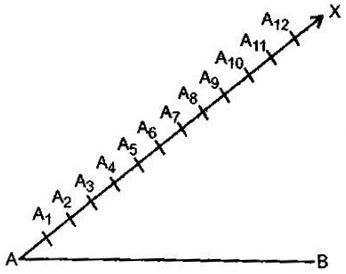According to the question, the minimum number of those points which are to be marked should be (Numerator + Denominator) i.e., 5 + 7 = 12

QUESTION: 3

### The angle of elevation of the top of a tower from a point on the ground and at a distance of 30m from its foot is 30°. The height of the tower is

Solution: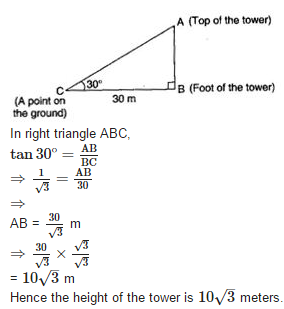QUESTION: 4

A die is thrown once. The probability of getting an even number and a multiple of 3 is

Solution:

Number of possible outcomes = {6} = 1

Number of Total outcomes = 6

∴ Required Probability = 1/6

QUESTION: 5

The distance of the point ( -3, 4) from the origin is

Solution:

Here, (x1,y1) = (−3,4) and (x2,y2)=(0,0)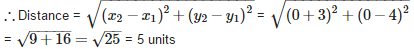QUESTION: 6

The area of a circle is 38.5 sq. cm. Its circumference is

Solution: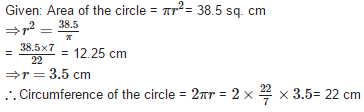QUESTION: 7

The cost of painting a cubical box of side 3m at the rate of Rs.2 per sq.m is

Solution:

Given: Side of the cube (a) = 3 m

∴ Surface Area of Cube = 6a2 = 6 x 3 x 3 = 54 sq. cm

Now, Cost of painting the cubical box of 1 sq. m = Rs. 2

∴ Cost of painting the cubical box of 54 sq. m = 54 x 2 = Rs. 108

QUESTION: 8

The value of ‘k’ for which the numbers x, 2x + k, 3x + 6 are in A.P. is

Solution:

If the numbers x,2x+k,3x+6 are in A.P.,

then 2x + k = x = 3x + 6 − 2x − k

⇒x + k = x + 6−k

⇒ 2k=6

⇒ k=3

QUESTION: 9

One of the roots of the quadratic equation a2x− 3abx + 2b= 0 is

Solution: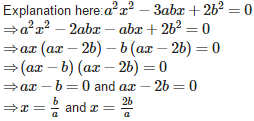QUESTION: 10

The next term of the A.P. √18, √32 and √50 is

Solution: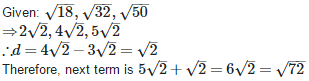QUESTION: 11

To construct a triangle similar to given ΔABC with its sides 8/5 of the corresponding sides of ΔABC, draw a ray BX such that ∠CBX is an acute angle and X is one the opposite side of A with respect to BC. The minimum number of points to be located at equal distances on ray BX is :

Solution:

According to the question, the minimum number of points to be located at equal distances on ray BX is 8.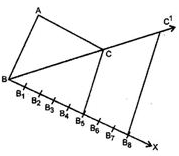QUESTION: 12

The length of tangent PQ, from an external point P is 24 cm. If the distance of the point P from the centre is 25 cm, then the diameter of the circle is

Solution: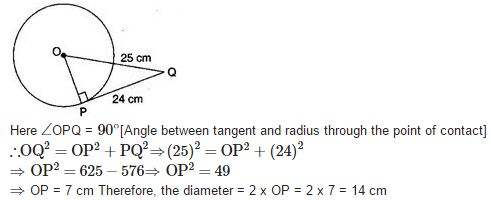QUESTION: 13

From a point on the ground which is 15m away from the foot of a tower, the angle of elevation is found to be 60°. The height of the tower is

Solution: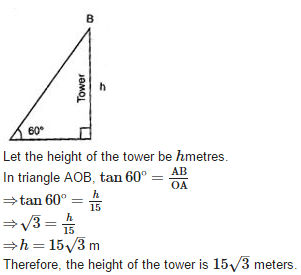QUESTION: 14

One card is drawn from a well shuffled pack of 52 cards. The probability of getting an ace is

Solution:

Number of possible outcomes = 4

Number of Total outcomes = 52

∴ Probability of getting an ace = 4/52=1/13

QUESTION: 15

The distance between the points ( – 1, – 5) and ( – 6, 7) is

Solution: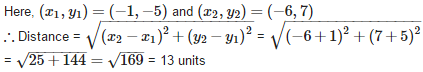QUESTION: 16

The circumference of a circle whose diameter is 4.2cm is

Solution:

Given: Diameter (d) = 4.2 cm

∴ Circumference = πd = 22/7×4.2 = 13.2 cm

QUESTION: 17

If the angles of a right angled triangle are in A.P. then the angles of that triangle will be

Solution:

Let the three angles of a triangle be a −d, a and a+d.
∴a − d + a + a + d=180°
⇒3a=180°
⇒a=60°
Therefore, one angle is of 60° and other is 90° (given).
Let third angle be x°, then
60° + 90° + x°=180°
⇒150° + x° =180°
⇒ x°= 180° − 150° = 30°
Therefore, the angles of the right angled triangle are 30°,60°,90°.

QUESTION: 18

the two numbers whose sum is 27 and their product is 182 are

Solution:

Let the one number be x then the other number will be (27−x) According to question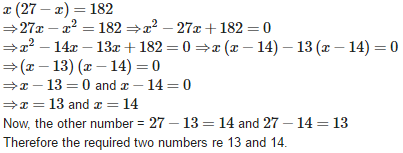QUESTION: 19

To divide a line segment AB in the ration 4 : 7, a ray AX is drawn first such that ∠BAX is an acute angle and then points A1,A2,A3,,…are located at equal distances on the ray AX and the point B is joined to :

Solution:

According to question, the point B is joined to A11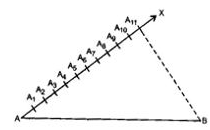QUESTION: 20

A solid cylinder of radius ‘r’ and height ‘h’ is placed over other cylinder of same height and radius. The total surface area of the shape so formed is

Solution: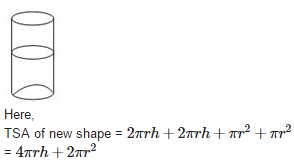QUESTION: 21

The length of the wire is 66m. The number of circles of circumference 13.2cm can be made from the wire is

Solution:

Given: Length of wire = 66 m = 6600 cm and Circumference = 13.2 cm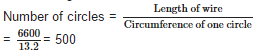QUESTION: 22

In the given figure, RQ is a tangent to the circle with centre O. If SQ = 6 cm and OR = 4 cm, then OR is equal to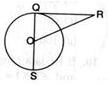Solution: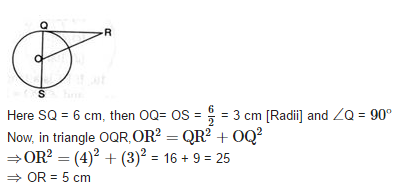QUESTION: 23

A box contains 3 blue balls, 2 white balls and 4 red balls. If a ball is drawn at random from the box, the probability of getting a white ball is

Solution:

Number of outcomes = 2

Number of total outcomes = 3 + 2 + 4 = 9

∴ Required Probability = 2/9

QUESTION: 24

From a point P on the level ground, the angle of elevation of the top of a tower is 30°. If the tower is 100m high, the distance between P and the foot of the tower is

Solution: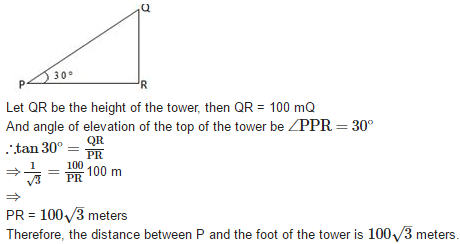QUESTION: 25

The distance between the points (a, b) and ( – a, b) is

Solution: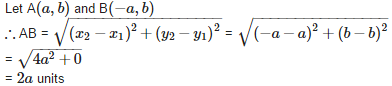QUESTION: 26

The common root of 2x+ x − 6=0 and x− 3x − 10=0 is

Solution: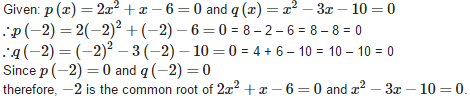QUESTION: 27

The list of numbers – 10, – 6, – 2, 2, ……….. is

Solution:

Given: list of numbers – 10, – 6, – 2, 2………..

Here, −6−(−10)=−6+10=4

−2−(−6)=−2+6=4

2−(−2)=2+2=4

Therefore, the list of given numbers is an A.P with common difference 4.

QUESTION: 28

To divide a line segment AB in the ration 2 : 5, first a ray AX is drawn, so that ∠BAX is an acute angle and then at equal distances points are marked on the ray such that the minimum number of these points is :

Solution:

According to the question, the minimum number of those points which are to be marked should be (Numerator + Denominator) i.e. , 2 + 5 = 7

QUESTION: 29

If two solid hemispheres of same base radius ‘x’ cm are joined together along their bases, then the CSA of the new solid formed is

Solution: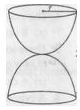If two solid hemispheres of same base radius ‘x’ cm are joined together along their bases, then the CSA of the new solid formed is 4πr= 4πx2.

QUESTION: 30

The perimeter of a protractor is

Solution:

Let radius of the protractor be r

∴ Perimeter of protractor = Perimeter of semicircle + Diameter of semicircle

⇒Perimeter of protractor = πr+2r

QUESTION: 31

In the adjoining figure, the measure Of PR is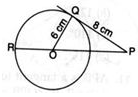Solution: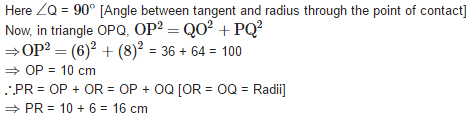QUESTION: 32

There are 8 defective items in a sample of 24 items. One item is drawn at random. The probability that it is a non defective item is

Solution:

Number of Non-defective items = 24 – 8 = 16

Number of possible outcomes = 16

Number of Total outcomes = 24

Probability of getting a Non-defective item = 16/24=2/3

QUESTION: 33

A ladder 14m long rests against a wall. If the foot of the ladder is 7m from the wall, then the angle of elevation is

Solution:

Let AC be the ladder of length 14 m and BC = 7 m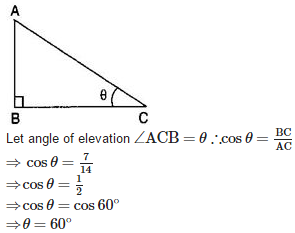QUESTION: 34

The values of ‘y’ for which the distance between the points (2, – 3) and (10, y) is 10 units is

Solution: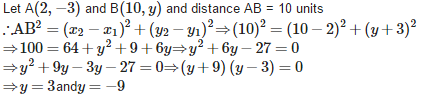QUESTION: 35

The roots of a quadratic equation x2 −4px + 4p− q2=0 are

Solution: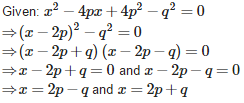QUESTION: 36

The first four terms of the sequence an= 2n + 3 are

Solution:

Given a= 2n + 3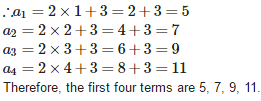QUESTION: 37

To divide a line segment PQ in the ratio 2 : 7, first a ray PZ is drawn so that ∠QPX is an acute angle and then at equal distances points are marked on the ray PX such that the minimum number of these points is :

Solution:

According to the question, the minimum number of those points which are to be marked should be (Numerator + Denominator) i.e., 2 + 7 = 9

QUESTION: 38

Two right circular cones have equal radii. If their slant heights are in the ratio 4 : 3, then their respective surface areas are in the ratio

Solution:

Let l1 and l2 be the slant heights of two cones respectively.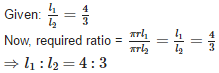QUESTION: 39

If the distance between the points (p, – 5) and (2, 7) is 13 units, then the value of ‘p’ is

Solution: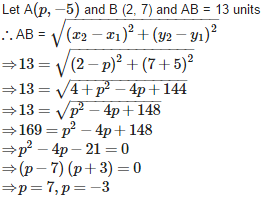QUESTION: 40

The radius of the circle whose area is equal to the sum of the areas of the two circles of radius 24cm and 7cm is

Solution: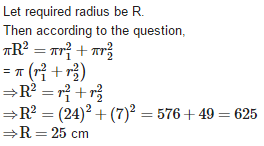QUESTION: 41

A rectangular piece of paper is 44cm long and 18cm wide. If a cylinder is formed by rolling the paper along its length, then the radius of the base of the cylinder is

Solution: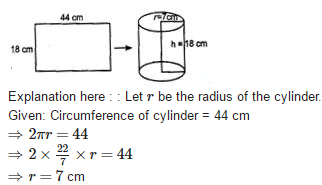QUESTION: 42

The product of two consecutive integers is 240. The quadratic representation of the above situation is

Solution:

Let one of the two consecutive integers be x then the other consecutive integer will be (x+1).

∴ According to question,

(x) × (x+1) = 240 ⇒ x(x+1) = 240

QUESTION: 43

If a, 7, b, 23, c are in A.P. then the value of ‘c’ is

Solution:QUESTION: 44

If the radius of the sphere is 2cm, then its curved surface area is

Solution: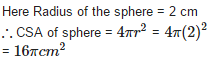QUESTION: 45

If ‘r’ is the radius of a circle, then its perimeter is given by

Solution:

If ′r′ is the radius of a circle, then its perimeter is given by 2πr.